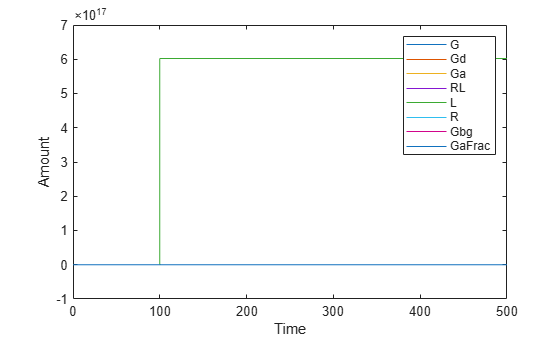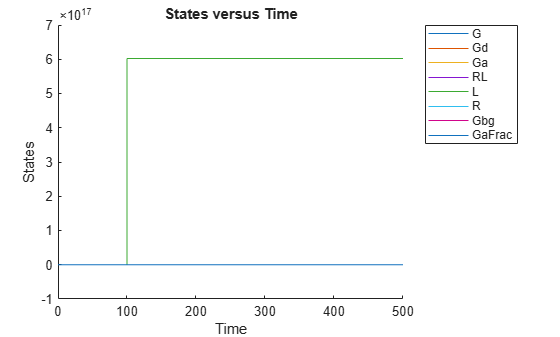## Simulate the Yeast Heterotrimeric G Protein Cycle

This example shows how to configure simulation settings, add an event to the model to trigger a time-based change, save, and plot the simulation results. This example uses the model described in Model of the Yeast Heterotrimeric G Protein Cycle to illustrate model simulation.

Load the `gprotein.sbproj` project, which includes the variable `m1`, a SimBiology® model object.

`sbioloadproject gprotein`

Set the simulation solver to `ode15s` and set a stop time of `500` by editing the `SolverType` and `StopTime` properties of the ```configset object``` associated with the `m1` model.

```csObj = getconfigset(m1); csObj.SolverType = 'ode15s'; csObj.StopTime = 500;```

Specify to log simulation results of all species.

`csObj.RuntimeOptions.StatesToLog = 'all';`

Suppose the amount of the ligand species `L` is 0 at the start of the simulation, but it increases to a particular amount at time = 100. Use `sbioselect` to select the species named `L` and set its initial amount to 0. Use `addevent` to set up the desired event.

```speciesObj = sbioselect(m1,'Type','species','Name','L'); speciesObj.InitialAmount = 0; evt = addevent(m1,'time >= 100','L = 6.022E17');```

Simulate the model.

`[t,x,names] = sbiosimulate(m1);`

Simulate the simulation results. Notice that the species L amount increases when the event is triggered at simulation time 100. Changes in other species do not show up in the plot due to the wide range in species amounts.

```plot(t,x); legend(names) xlabel('Time'); ylabel('Amount');```To see the changes of other species, plot without the species L (the 5th species) data.

```figure plot(t,x(:,[1:4 6:8])); legend(names{[1:4 6:8]}); xlabel('Time'); ylabel('Amount');```Alternative to storing simulation data in separate outputs, such as `t`, `x`, and `names` as above, you can store them all in a single `SimData object`. You can then use `selectbyname` to extract arrays containing the simulation data of your interest.

```simdata = sbiosimulate(m1); sbioplot(simdata);```Expand Run 1 to see the names of species and parameter that are plotted.

```simdata_noL = selectbyname(simdata, {'Ga','G','Gd','GaFrac','RL','R'}); sbioplot(simdata_noL);```##### SupportGet trial now